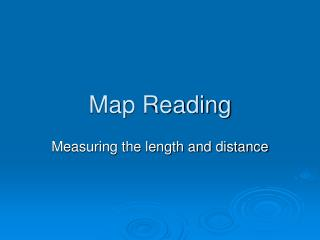DownloadDownload PresentationDownload Presentation- - - - - - - - - - - - - - - - - - - - - - - - - - - E N D - - - - - - - - - - - - - - - - - - - - - - - - - - -
##### Presentation Transcript

1. Map Reading Measuring the length and distance

2. Measuring straight line distance between two points. • Join the two places with a straight line. • Measure the length between them on the map with a ruler. 5.5 cm • Identify the scale of the map: 1cm to 3.2 km • Do the calculation for the answer: 3.2 km X 5.5 = 17.6km

3. Measuring straight line distance between two points. • Join the two places with a straight line. • Measure the length between them on the map with a ruler. • Identify the scale of the map • Do the calculation for the answer: Scale X Distance on the map

4. Finding the actual ground distance of a curved line by using a piece of paper. • Use a piece of paper with straight edge. • Divide the road into different parts. Each part could be measured as a straight line.

5. Finding the actual ground distance of a curved line by using a piece of paper. • Place the edge of the paper against the first part of the road. • Use to tip of a pencil to pin the paper at the end of the first part , the twist the paper till attaches to another straight part.

6. Finding the actual ground distance of a curved line by using a piece of paper. • Same method is applied to measure the remaining straight

7. Finding the actual ground distance of a curved line by using a piece of paper. • Place the paper along a ruler and obtain the map distance of the road in cm.

8. Finding the actual ground distance of a curved line by using a piece of paper. • With reference to the linear scale, measure the actual ground distance of the road from Perth to Sydney. • Do the calculation: the scale is 1.5 cm to 500km. So the actual ground distance of the road is (10.7/1.5 X 500m)=3567km